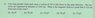# Magnetic Field, Parallel wires carrying current

jegues

## Homework Statement

See figure attached.

## The Attempt at a Solution

i = 5A, d=8cm, R=5cm.

Okay so we will have a two 3,4,5 triangles back to back from the center of each wire to the point in question. Since the currents are in the same direction, the magnetic field will point in the same direction and add together.

$$B = \frac{\mu _{0}i}{\pi R} = 40 \mu T$$

The answer is given as 24uT.

What am I doing wrong?

#### Attachments

•Q6.jpg
15 KB · Views: 487

da_nang
Since the currents are in the same direction, the magnetic field will point in the same direction and add together.
There's your problem. Parallell wires with currents in the same direction create magnetic fields that oppose each other in the area between the wires. Also remember that there's an angle between the magnetic fields at that point. Use vectors and divide them into components. You'll notice that the vertical components cancels out, but the horizontal ones add together. That's the answer you're looking for.

Last edited:
jegues
There's your problem. Parallell wires with currents in the same direction create magnetic fields that oppose each other in the area between the wires. Also remember that there's an angle between the magnetic fields at that point. Use vectors and divide them into components. You'll notice that the vertical components cancels out, but the horizontal ones add together. That's the answer you're looking for.

I don't think they do make fields that oppose each other. Suppose both wires a sticking out of the page, if we stick our right thumb in that direction the direction in which our fingers curl will be the direction of the magnetic field, thus they are in the same direction.

Edit: they only oppose each other in the y directions, their x components add together.

So if I double that x component of one of them I'll get the answer.

Last edited: